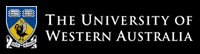School of Computer Science and Software Engineering

# School of Computer Science and Software Engineering

## CITS4406 Problem Solving and Programming

#### Labsheet 4: Strings and Lists

This labsheet is in three parts: a number of review exercises that reinforce concepts from the lectures; an important problem-solving technique, motivated by its use in solving a larger-sized problem representative of the types of problems that can be solved with computers; and some basic list operations that will be useful in the project

#### Review Exercises

1. Assuming that the variable data refers to the string "myprogram.exe", write an expression that (a) extracts the substring "gram", (b) truncates the extension ".exe", (c) extracts the character at the middle position.

2. An acronym is a word formed by taking the first letters of each word in a a phrase and making a new word from that (e.g. RAM is an acronym for random access memory). Write a program that allows the user to type ina phrase and then outputs the acronym for that phrase. Note the acronym should all be in upper case, even if the words in that phrase are not capitalised.
3. Write a program to calculate teh average word length in a phrase entered by the user.
4. Assuming that the variable data refers to the string "Python rules!", use a string method to (a) obtain a list of the words in the string, (b) convert the string to uppercase, (c) locate the position of the string "rules", (d) replace the exclamation point with a question mark.

5. Write a code segment that opens a file for input and prints the number of 4-letter words in the file.

6. A bit shift is a procedure that takes a binary string and moves the digits either left or right, wrapping digits around as required; e.g. a left shift of 1 applied to 10011 gives 00111, and a left-shift of 2 gives 01110. Write a code segment that takes a string s, an integer k, and a Boolean b, and that prints s shifted by k, left if b if True, and right otherwise.

7. Write a code segment that inputs a string and replaces all occurrences of "ten" with "ten (10)". The code should work for upper-case and lower-case.

8. Write a code segment that prints the names of all of the items in the current working directory.

9.Write a script copyfile.py that prompts the user for the names of two text files, inputs the contents of the first file, and writes them to the second file.

#### Problem Solving Technique: Abstraction and Generalization

Abstraction is the problem solving technique of examining a model of a situation instead of the situation itself. The model can be a much simplified version of the problem so that the unecessary details do not get in the way.

PROBLEM SOLVING QUESTION 4.1) Count how many people are in your lab class, or in a typical CS lab. What is the probability that two people in such a lab will share the same birthday (month and day of the month)? Use random numbers and a reasonable number of repeated simulations of a lab class full of birthdays to estimate this probability. Did you use actual dates or some abstraction of the date?

Related to Abstraction is the technique of Generalisation. This is the idea of solving a more general problem.

PROBLEM SOLVING QUESTION 4.2) In lab 3, we developed an algorithm for converting from binary to decimal. You can generalize this algorithm to work for a representation in any base. Instead of using a power of 2, this time you use a power of the new base. Also, you can use digits greater than 9, such as A… F, when they occur. Write a function with parameters string and integer base parameters and returns the decimal (base 10) value of the string. For simplicity, consider only base values upto 16.

#### Basic list operations

1. Lists are used to hold a sequence of elements, much like a string is a sequence of characters. What is the difference between a String and a sequence of characters?
2. Given the initial statements:
``` import string s1 = [2,1,4,3] s2 = ['c','a','b'] ```
What is the effect of the following expressions:
1. `s1 + s2`
2. `3 * s1+ 2 * s2`
3. `s1`
4. `s1[1:3]`
5. `s1.append(6)`
6. `s1.sort()`
3. Write a program to create a list containing the first n elements of the Fibonacci sequence. The Fibonacci sequence starts:
1, 1, 2, 3, 5, 8, 13, 21, ...
where each successive number os the some of the two previous numbers.

# The University of Western Australia

## University information

CRICOS Code: 00126G